# NCERT Solutions for Class 5 Maths Tenths And Hundredths## myCBSEguide App

CBSE, NCERT, JEE Main, NEET-UG, NDA, Exam Papers, Question Bank, NCERT Solutions, Exemplars, Revision Notes, Free Videos, MCQ Tests & more.

NCERT solutions for Class 5 Mathematics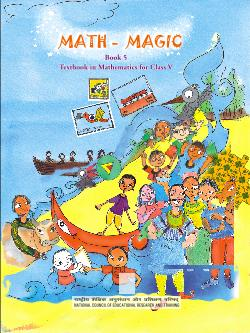## NCERT Solutions for Class 5 Maths Tenths And Hundredths

###### 1. Length of the nail …. Cm and …. Mm or …. Cm.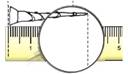Ans. Length of the nail 2 cm and 9 mm or 2.9 cm.

###### 2. The length of this lady’s finger (bhindi) is …. cm and …. Mm. We can also write it as .. mm.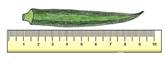Ans. The length of this lady’s finger is 8 cm and 3 mm. We can also write it as 8.3 cm.

###### 3. Using the scale on this page find the difference in length between candle 1 and candle 3?Ans.

 Length of Length in cm and mm Length in cm. Candle 1 2 cm and 9 mm 2.9 cm Flame 1 1 cm and 3 mm 1.3 cm Candle 2 4 cm and 9 mm 4.9 cm Flame 2 1 cm and 6 mm 1.6 cm Candle 3 6 cm and 0 mm 6 cm Flame 3 1 cm and 5 mm 1.5 cm

NCERT Solutions for Class 5 Maths Tenths And Hundredths

###### 4. Our Eyes Get Confused?

Which line is longer? A or B? Measure each line and writes how long it is in centimetres. How good is your guess?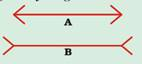Ans. I think line B is longer than A. On Measuring: line (A) = 4.6 cm and Line (B) = 4.6 cm.

My guess is incorrect.

###### 5. Which line is longer? C or D? Measure each line. How good is your guess?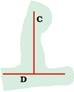Ans.  Line (C) appears to be longer than the line (D). On measuring: Line (C) = 3.2 cm and Line (D) = 3.2 cm. My guess in incorrect.

NCERT Solutions for Class 5 Maths Tenths And Hundredths

###### 6. At the market

(a) How many paise does a matchbox cost?

Ans. A matchbox costs 50 paise.

(b) How many match-box can be got for Rs. 2.50?

Ans. Five matchboxes can be bought for Rs. 2.50.

(c) How many rupees does the soap cost?

Ans. A soap costs Rs. 8.75.

(d) Arun wanted to buy a soap. He has a five-rupees coin. 2 one-rupee coins and 4 half-rupee coin. Write in rupees what money he will get back?

Ans. Total money with Arun = 1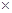Rs. 5 + 2Re 1 + 4Re. 0.5

= Rs. 5 + Rs. 2 + Rs. 2 = Rs. 9

Cost of a soap = Rs. 8.75

Arun will get back = Rs. 9 – Rs. 8.75

= 900 paise – 875 paise

= 25 paise = Re 0.25

(e)(1) An egg costs two and a half rupees. How much will one and a half dozen cost?

Ans. (1) One dozen =12 pieces

One and a half dozen = (12+6) = 18 pieces

Cost of one egg = Rs. 2.50

Cost of 18 eggs = Rs. (182.50)

= Rs. 182 + Rs. 180.50

= Rs. 36 + Rs. 9 = Rs. 45

(2) How many pens can Kannan buy? How much money is left?

Ans. (2) Money left after purchase of one and a half dozen eggs

= Total money – Money spent

= Rs. 60 – Rs. 45

= Rs. 15

Cost of a pen = Rs. 6.50

Since 1Rs. 6.50 = Rs. 6.50, 2Rs. 6.50 = Rs. 13

3Rs. 6.50 = Rs. 19.50

Money left with Kanna = Rs. 15- Rs. 13 = Rs. 2

(f) The price of two pens is Rs …… Can she buy two pens?

Ans. Price of 1 pen = Rs. 6.50

Price of 2 pens = Rs. (26.50) = Rs. 13

So, she can buy 2 pens with rupees 13.

NCERT Solutions for Class 5 Maths Tenths And Hundredths

###### 7. Colourful Design: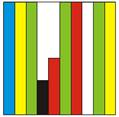(a) What part of this sheet is coloured blue?

Ans. (a)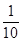part of the given sheet is coloured blue.

(b) Which part of the sheet is green?

Ans.(b)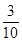part of the given sheet is green.

(c) Which colour covers 0.2 of the sheet?

Ans. (c) Black colour covers 0.2 of the given sheet.

NCERT Solutions for Class 5 Maths Tenths And Hundredths

###### 8.  Now look at the second sheet. Each strip is divided into 10 equal boxes. How many boxes are there in all?

(a) Is each box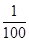part of the sheet?

Ans. (a) Yes, each box ispart of the sheet.

(b) How many blue boxes are there?

Ans. (b) There are 10 blue boxes.

(c) Is blue equal to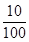of the sheet? We saw that blue is also equal toof the sheet. We wrote it as 0.1 of the sheet.

Ans. (c) Yes, blue boxes are equal toof the sheet.

(d) Can we say== 0.10 = 0.1 ?

Think: Can we write ten paise is 0.1 of a rupee?

Ans. (d) Yes, we can say== 0.10 = 0.1.

(e) How many boxes are red? What part of the sheet is this?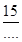Can we also write it as 0.15 of the sheet?

Ans. There are 15 red boxes. They are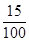part of the sheet.

It can also be written as 0.15 of the sheet.

(f) Now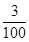of the sheet is black. We can say 0. ……. Is black.

Ans. Nowof the sheet is black. We can say 0.03 sheet is black.

(g) How many white boxes are there in the sheet?

Ans. There are 22 white boxes in the sheet.

(h) What part of the second sheet is white?

Ans.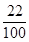part of the second sheet is white.

NCERT Solutions for Class 5 Maths Tenths And Hundredths

###### 9. Sports Day The school at Malappuram has its sports day. The first five children in the Long Jump are: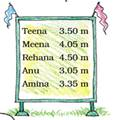(a) Who is the winner in the long jump?

Ans. (a) Rehana was the winner.

(b) Write the names of the I , II and III winners on this stand.

Ans.  I is Rehana, II is Meena and III is Teena.

(c) Do you remember that 1 metre = 100 centimetere? So one centimeter isof a metre. We also writes 1 cm as ….. m.

Ans. (c) 1 cm as 0.10 m.

NCERT Solutions for Class 5 Maths Tenths And Hundredths

###### 10.  Money from different countries.

Have you seen any notes or coins used in any other country? Shivam Bank has a chart to show us how many Indian rupees we can get when we change the money of different countries.

 Country Money Changed into Indian rupees Korea Won 0.04 Sri Lanka Rupee (SL) 0.37 Nepal Rupee 0.63 Hong Kong Dollar (HK) 5.10 South Africa Rand 5.188 Saudi Riyal 10.52 China Yuan 5.50 U.A.E Dirham 10.80 U.S.A Dollar 39.70 Germany Euro 58.30 England Pound 777.76

(This is the rate on 15-2-2008)

(A) The money of which country will cost the most in Indian Rupees?

Ans. England’s money will cost the most in Indian Rupees.

(B) Mithun’s uncle in America had sent hin 10 USA dollars as a gift. Mithun used 350 rupees for a school trip. How much money was left with him.

Ans. Money received by Mithun = 10 USA Dollars

= 10Rs. 39.70

= 10Rs. 39 + 1070 paise

= Rs. 390 + 700 paise

= Rs. 390 + Rs. 397

Money spend on school trip =Rs. 350

Money left with Mithun = Rs. 397- Rs. 350 = Rs. 47

(C) Majeed’s father is working in Saudi Arabia. He gets 1000, Saudi Riyal as salary. Arun’s father who is working in Sri Lanka gets 2000 Sri Lankan Rupees. Who gets more Indian Rupees.

Ans. Majeed’s father salary = 1000 Saudi Riyal

= 1000Rs. 10.52

= 1000Rs. 10 + 100052 paise

= Rs. 10000 + 52000 paise

= Rs. 10000 + Rs. 520 = Rs. 10520

Arun’s father salary = 2000 Sri Lankan Rupees

= 2000Rs. 0.37

= 200037 paise

= 74000 paise = Rs. 740

(D) Leena’s aunty brought a present for her from China. It cost 30 Yuan. Find what it costs in Indian rupees.

Ans. Cost of the present = 30 Yuan

= 30Rs. 5.50

= 30Rs. 5 + 3050 paise

= Rs. 150 + Rs. 15 = Rs. 165

(E) Astha wants some Hong Kong Dollars and Won.

(1) How many won can Hong Kong Dollars and Won.

Ans. Since 1 won = Re. 0.04

Multiply both sides by 100, we get

1001 Won = 100Re. 0.04

= 1004 paise

= 400 paise = Rs. 4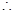For Rs.4 Astha can have 100 Won

And, for Rs.400, she can have 100100 = 10000 won

(2) How many Hong Kong Dollars can she changes for Rs. 508?

Ans. Since 1 Dollar (HK) = Rs. 5.10For Rs. 508, Astha can have 99 Dollar and have left with Rs. 508- Rs. 504.90 i.e. Rs. 3.10 in hand.

NCERT Solutions for Class 5 Maths Tenths And Hundredths

###### 11. Kiran went shopping with Rs. 200. Look at the bill. The shopkeeper forgot to put the point correctly in the prices. Put the point in the correct place and find out the total amount of the bill.
 Item Quantity Price (Rupees) Soap 1 12.50 Green gram 1 kg 50.25 Tea 250 gm 27.25 Coconut Oil 1 Litre 60.00 Total

Ans. The correct bill as under:

 Item Quantity Price (Rupees) Soap 1 12.50 Green gram 1 kg 50.25 Tea 250 gm 27.25 Coconut Oil 1 Litre 60.00 Total 150.00

NCERT Solutions for Class 5 Maths Tenths And Hundredths

###### 12. Which city is cool?

The temperature in each city was noted at 3pm on 16 January, 2008 as tabulated below:

(a) Which place had the highest temperature at 3 pm? Which place is the coolest at that time?

Ans. (a) Mumbai had the highest temperature at 3 pm. Srinagar had the coolest temperature at 3 pm.

(b)  How much higher is the temperature in Mumbai from that in Srinagar?

Ans. (b) The temperature in Mumbai is higher from that in Srinagar by 35.1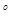C. 8.1C = 27C

(c) How many degrees will the temperature need to rise for it to reach 40C in Thiruvananthapuram?

Ans. (c) There should be a rise of 40C- 33.5C = 6.5C on temperature for it to reach 40C in Thiruvananthapuram.

(d) How much lower is the temperature of Kolkata from that in Chennai?

Ans. (d) The temperature in Kolkata is lower than in Chennai by 29.9C – 26.6C = 3.3C.

(e) The temperature in these cities was also noted at 3 am on the same day, Look at the table and answer the questions.

Q. Which place had the lowest temperature at 3 am? Imagine yourself to be there and describe how it would feel?

Ans. Srinagar had the lowest temperature at 3 am. It was very much cold and the water n pipes froze into ice. We had worn heavy wollen clothes and had also taken the help of fire

Q. What is the difference between the temperatures at 3 pm and 3 am in Chennai?

Ans. The difference between the temperatures at 3 pm and 3 am in Chennai is 29.9C – 21.1C = 8.8C.

## NCERT Solutions for Class 5 Mathematics Chapter 10 Tenths And Hundredths

NCERT Solutions Class 5 Maths PDF (Download) Free from myCBSEguide app and myCBSEguide website. Ncert solution class 5 Maths includes text book solutions from Class 5 Maths Book . NCERT Solutions for CBSE Class 5 Maths have total 14 chapters. 5 Maths NCERT Solutions in PDF for free Download on our website. Ncert Maths class 5 solutions PDF and Maths ncert class 5 PDF solutions with latest modifications and as per the latest CBSE syllabus are only available in myCBSEguide.

## CBSE app for Students

To download NCERT Solutions for Class 5 Maths, Social Science Computer Science, Home Science, Hindi English, Maths Science do check myCBSEguide app or website. myCBSEguide provides sample papers with solution, test papers for chapter-wise practice, NCERT solutions, NCERT Exemplar solutions, quick revision notes for ready reference, CBSE guess papers and CBSE important question papers. Sample Paper all are made available through the best app for CBSE students and myCBSEguide website.### 7 thoughts on “NCERT Solutions for Class 5 Maths Tenths And Hundredths”

1. PRACTICE TIME – MATCH THESE ON
PAGE NO. 140 IS MISSING

2. Where is 136 page? I wanted 136 page .
Well, its a good app.

3. thank

4. nice solution

5. This website is useful for children

6. This website is good for children

7. You left this question: In Bhopal?